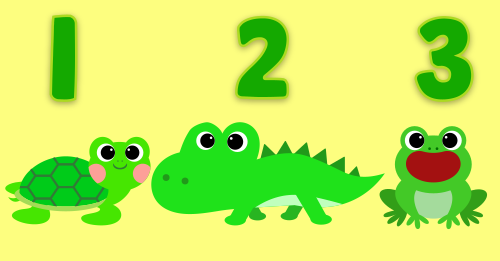Let's count these dragon fruits together!Can you count these school buses?Let's do some simple calculations.Can you count these bicycles?Can you count these lemons?Let's count these raspberries and melons together!Let's do some simple calculations.Can you count these oranges?Do you know what you get when two colors are mixed into one?Can you tell the first letters of these words?Can you identify numbers up to 10?Can you tell the order of these animals?Which one is bigger?Let's count hippos together!Can you count these snakes please?Can you find the correct capital letter?Let's do some simple calculations.Kids learn colors and numbers with balloonsDo you know the names of the fruits?Do you know the color of these airplanes?